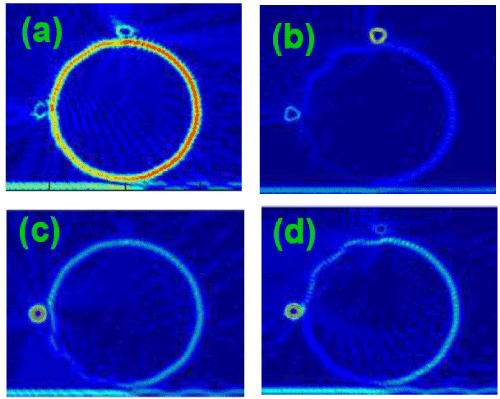Figure 5: FDTD simulation of light intensity in the microring sensing system with two sensing objects having r=1micron. (a) light frequency f=191 THz that is resonant in the cavity with two SOs n=2.0. The SOs have no eigenmodes resonant with light frequency; (b) light frequency f=192.2 THz that is resonant with an eigenmode of two identical SO with n=3.0; (c) light frequency f=188.6 THz which is resonant with an eigenmode of SO with n=3.4 but not resonant with the other SO n=3.0, and (d) light frequency f=194.6 THz that is resonant with SO n=3.6 but not resonant with SO n=3.0.
Goto home»